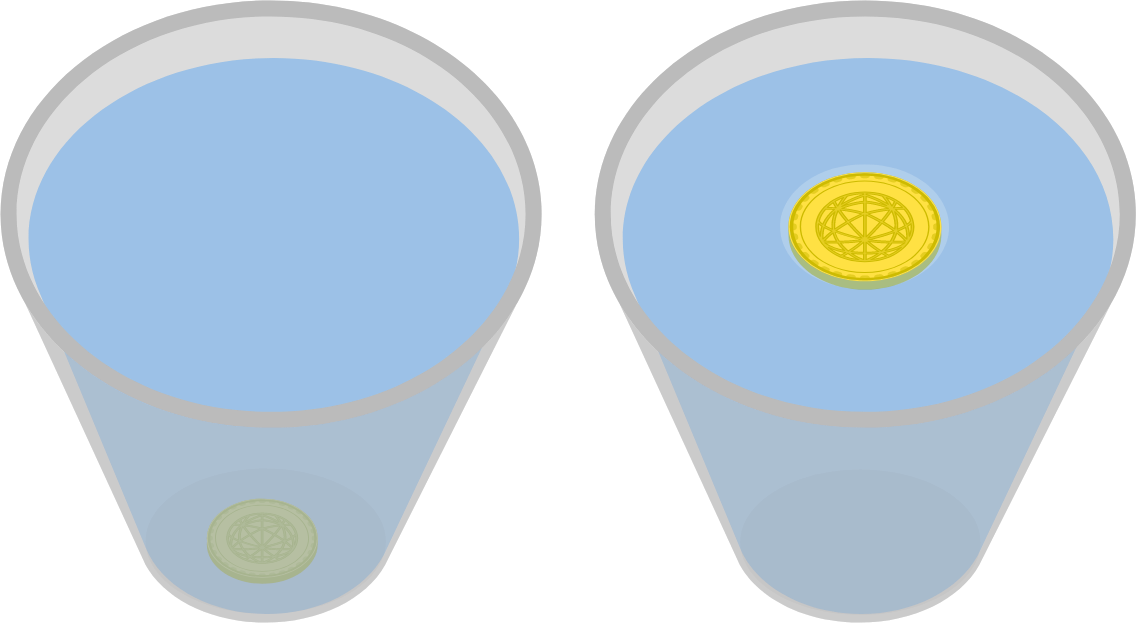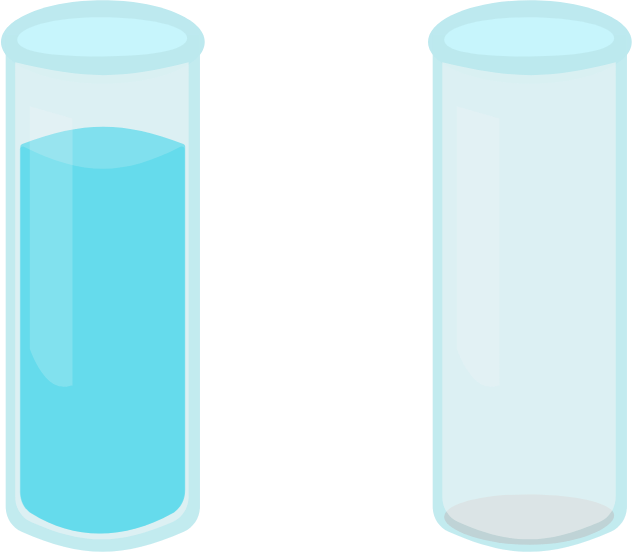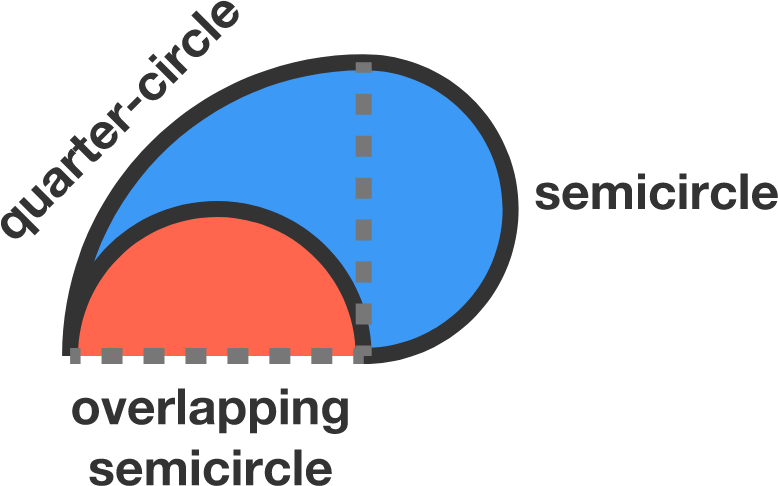# Problems of the Week

Contribute a problem

# 2018-03-12 BasicRakesh’s spam filter predicts whether or not an email is spam. It is 98% accurate at identifying either type of email, and he knows that 1% of the emails sent to him are spam.

Which is more likely to be true if he receives an email that his filter predicts is spam?

If you drop a coin in water, it sinks. However, if you slowly lower the coin onto the surface of the water so that its flat bottom is parallel to the surface of the water, it floats. Why does this happen?One glass is full of water and the other is empty.

When a knife lightly strikes the top of each glass, which glass will produce a lower pitch?A circle is divided into four identical regions constructed out of overlapping quarter-circles and semicircles.

If the circumference of the circle is 120, then what is the perimeter of each region?The construction of each region is shown here.Suppose you have a $2 \times 2 \times 2$ cube and you can color each of the 24 little squares one of $\color{#D61F06}\text{f}\color{#20A900} \text{o}\color{#3D99F6} \text{u}\color{#EC7300} \text{r}$ colors.

Is it possible to paint the cube so that the following holds true?

• None of the 3 squares meeting at a corner are the same color.
• None of the 4 squares meeting along an edge are the same color.
• None of the 4 squares on any face are the same color.
×